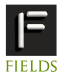June 24-28, 2012 The 2012 Annual Meeting of the Canadian Applied and Industrial Mathematics SocietyToronto Information Contact Us programs(PUT_AT_SIGN_HERE)fields.utoronto.caMini Symposium on Recent Advances in Computational Methods for PDEs with Applications

by Paul Muir
Saint Mary's University
Coauthors: Christina Christara, University of Toronto,

Mathematical models in a great majority of applications involve the numerical solution of partial differential equations (PDEs). Essentially all such models are sufficiently complicated that the associated PDEs typically must be solved using sophisticated numerical algorithms. This mini-symposium will bring together researchers who will discuss recent developments in new computational algorithms for the numerical solution of PDEs including high-order collocation methods in one and two spatial dimensions, domain decomposition algorithms, and symplectic finite-difference time-domain schemes, applied to problems including modelling of intracellular signalling pathways (mathematical biology), pricing of American options (computational finance), and the numerical solution of Maxwell's equations (computational electromagnetism).

Confirmed speakers:

Zhi Li, Saint Mary's University
B-spline collocation software for 2D Parabolic PDEs, with application to Cell Biology

In this talk, we describe new software, BACOL2D, for solving two dimensional time-dependent parabolic partial differential equations (PDEs), defined over a two dimensional rectangular region. The numerical solution is represented as a bi-variate piecewise polynomial (using tensor product B-spline bases) with unknown time dependent coefficients. These coefficients are determined by imposing collocation conditions, i.e., by requiring the numerical solution to satisfy the PDE at a number of points within the spatial domain. This leads to a large system of time-dependent
differential algebraic equations (DAEs), which we solve using the high quality DAE solver, DASPK 2.0. BACOL2D is natural extension of the one-dimensional PDE solver BACOL and many of the algorithms employed in BACOL are applicable to BACOL2D. We also describe an algorithm for a fast block LU solver with modified alternate row and column elimination with partial pivoting for the treatment of the almost block diagonal linear systems that arise during the numerical solution of the DAEs. We will also briefly consider numerical results to demonstrate convergence rates for the collocation solution and, in particular, the existence of superconvergent points that may be useful for error estimation. We also demonstrate the use of BACOL2D to solve a 2D cell biology model.

Duy Minh Dang, University of Waterloo
Adaptive and high-order methods for pricing American options

We develop space-time adaptive and high-order methods for valuing American options using a partial differential equation (PDE) approach. The linear complementarity
problem arising due to the free boundary is handled by a penalty method. Both finite difference and finite element methods are considered for the space discretization of the PDE, while classical finite differences, such as Crank-Nicolson, are used for the time discretization. The high-order discretization in space is based on an optimal finite element collocation method, the main computational requirements of which are the solution of one tridiagonal linear system at each time step, while the resulting errors at the gridpoints and midpoints of the space partition are fourth-order. To control the space error, we use adaptive gridpoint distribution based on an error equidistribution principle. A time stepsize selector is used to further increase the efficiency of the methods. Numerical examples show that our methods converge fast and provide highly accurate options prices, Greeks, and early exercise boundaries.

Dong Liang, York University,
New Energy Conservation Properties and Energy-Conserved S-FDTD Schemes for Maxwell's Equations

Computational electromaganetics has been playing a more and more important role in many areas of electromagnetic industry, such as radio frequency, microwave, integrated optical circuits, antennas, and wireless engineering, etc. It is of special importance to develop efficient high-order methods for effective and accurately simulating propagation of electric and magnetic waves in large scale field and long time duration. However, most previous ADI or splitting schemes break the energy conservation of elctromegnetic waves. In this talk, I will first give new energy conservation properties of electromagnetic waves in lossless medium, and then provide our newly developed energy-conserved S-FDTD schemes for Maxwell's equations. Both theoretical analysis and numerical experiment will be presented to show the efficiency of the new schemes.

Hans De Sterck, University of Waterloo
Global approximation of singular capillary surfaces: asymptotic analysis meets numerical analysis

Singular capillary surfaces in domains with sharp corners or cusps are well studied and the asymptotic series approximation of the solution is known. However, the asymptotic series approximation is only valid in a sufficiently small neighbourhood of the singularity, and we wish to obtain a global approximation of the solution through finite element approximation. Yet it is also known that the singularity of the solution spoils the accuracy of standard finite element approximations, which cannot reproduce the singularity accurately. We show that an accurate numerical approximation can be obtained through an appropriate change of variable combined with a change of coordinates, motivated by the known
asymptotic behaviour. Using this accurate numerical approximation methodology, we can numerically confirm the validity of the known asymptotic expansions in great detail, and we can make two conjectures on asymptotic behaviour of singular capillary surfaces at a cusp for two open cases.